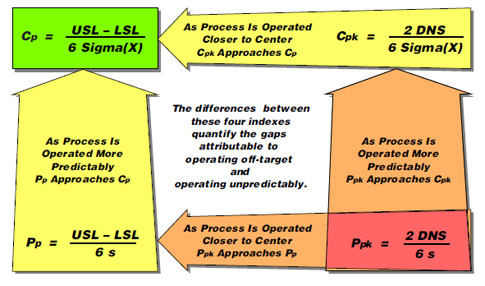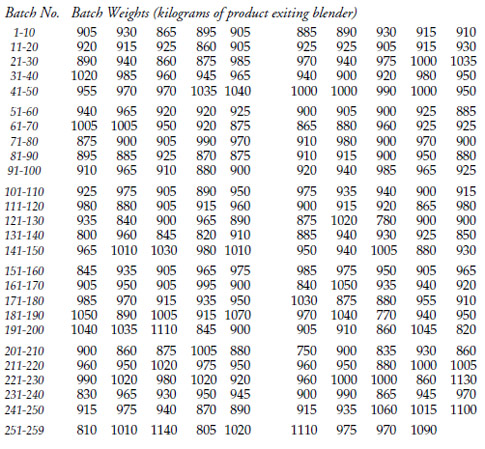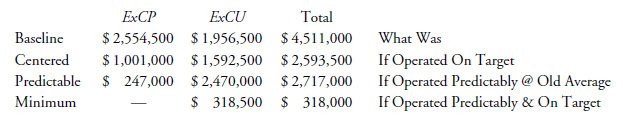#Quality Digest

Featured Product
This Week in Quality Digest Live
Six Sigma Features
Douglas C. Fair
Part 3 of our series on SPC in a digital era
Scott A. Hindle
Part 2 of our series on SPC in a digital era
Donald J. Wheeler
Part 2: By trying to do better, we can make things worse
Douglas C. Fair
Introducing our series on SPC in a digital era
Donald J. Wheeler
Part 1: Process-hyphen-control illustrated
Six Sigma News
How to use Minitab statistical functions to improve business processes
Sept. 28–29, 2022, at the MassMutual Center in Springfield, MA
Elsmar Cove is a leading forum for quality and standards compliance
Is the future of quality management actually business management?
Too often process enhancements occur in silos where there is little positive impact on the big picture
Collect measurements, visual defect information, simple Go/No-Go situations from any online device
Good quality is adding an average of 11 percent to organizations’ revenue growth
Floor symbols and decals create a SMART floor environment, adding visual organization to any environment
A guide for practitioners and managersBio

Six Sigma

## The Gaps Between Performance and Potential

### Using the effective costs of production and use

Published: Wednesday, September 1, 2010 - 05:00

In my August column, “How to Turn Capability Indexes Into Dollars,” I defined the effective cost of production and use and showed how it can be obtained directly from the capability and performance indexes. In this column, I will show how these indexes can be used to estimate the benefits to be obtained from different improvement strategies.

### Capability and performance indexes

The capability ratio, Cp, compares the space available within the specifications with the space required by the process. The performance ratio, Pp, compares the space available within the specifications with the space used by the process in the past. The only difference between these two ratios is the manner in which the denominator is computed. The capability ratio uses a within-subgroup measure of dispersion, Sigma(X), while the performance ratio uses a global standard deviation statistic, s. When a process is operated predictably, these two measures of dispersion tend to converge, and the two ratios will be quite similar. However, when a process is operated unpredictably the global measure of dispersion will be inflated relative to the within-subgroup dispersion, which will deflate the performance ratio.

In a similar manner, the centered capability ratio, Cpk, compares twice the distance to the nearest specification, 2 DNS, with the space required by the process, while the centered performance ratio, Ppk, compares 2 DNS with the space used in the past. Thus, these four index numbers are related in the manner shown in figure 1.

The top tier in figure 1 represents the actual capability of a predictable process, or the hypothetical capability of an unpredictable process. The bottom tier represents the actual past performance of a process.

The left side of figure 1 describes the potential or the performance of a process that is centered at the midpoint of the specifications, while the right side describes how the potential or performance suffers when the process is not centered within the specifications.

When a process is operated predictably and on target, these four indexes will be four estimates of the same thing. For example, the Tokai Rika data given in my August column came from a process that was being operated predictably and on target. Those data have index numbers of Cp = 2.26, Cpk = 2.21, Pp = 2.40, and Ppk = 2.35.Figure 1: Capability and performance indexes

When a process is operated predictably but is not centered within the specifications, there will be a discrepancy between values on the right and left sides in figure 1.

When a process is being operated unpredictably, the indexes in the bottom tier of figure 1 will be substantially smaller than the indexes in the top tier.

Finally, when a process is operated unpredictably and off target, the four indexes will be estimates of four different quantities. Although the capability ratio will be the best-case value, the centered performance ratio will be the worst-case value, and the gap between these two values will define the opportunities for improvement connected with operating the process up to its full potential.

### The batch weight data

The data in figure 2 are the weights of 259 sequential batches. The specifications are 900 kg to 1,100 kg, with a target value of 1,000 kg. The process behavior chart is shown in figure 3.

The average of the first 59 moving ranges is 25.8, which results in a Sigma(X) value of 22.9. Thus, the capability ratio is found to be 1.46. The average of the first 60 values is 938.1. This results in a DNS value of 38.1, and a centered capability ratio of 0.55. The discrepancy between 1.46 and 0.55 summarizes the extent to which this process is off target.

The global standard deviation statistic for the 259 batch weights is s = 61.3. Thus, the performance ratio is 0.54. The average of all 259 values is 937. This results in a DNS value of 37 and a centered performance ratio of 0.20.Figure 2: Batch weight dataFigure 3: XmR chart for the batch weight data

The discrepancy between Cp = 1.46 and Pp = 0.54 quantifies the extent to which this process is being operated unpredictably.

### Baseline costs of production and use

We use Pp and Ppk to find a baseline value for the effective cost of production and use. While it may be difficult to predict what an unpredictable process is going to do in the future, we can be reasonably sure that spontaneous improvement is highly unlikely. If we do not do anything, the future is unlikely to be any better than the past, and it could be worse. Therefore, by characterizing the baseline costs of production and use for the past, we have a reasonable value for purposes of comparison. In this case, all nonconforming product is scrapped, and the cost of scrap is close to the nominal cost of production. Using the tables provided in my new book, Reducing Production Costs (SPC Press, 2010), we find an excess cost of production of 0.394, an excess cost of use of 0.301, and an effective cost of production and use of 1.694. At a nominal cost of \$500 per batch, and with an annual volume of 13,000 batches, this works out to a baseline annualized excess cost of:

Baseline Annualized Excess Cost = 0.694 × \$500 × 13,000 = \$4,511,000

If the company could wave a wand and make things perfect, this is the potential amount it could save during the course of a year. Because perfection is unlikely to occur, this \$4.5 million represents the opportunity pool that exists for improvement projects. (Such opportunity pools are sometimes known as “entitlements.”) In this case, because the producer and the cus­tomer are separate departments in the same company, it is appropriate to use both the excess costs of production and the excess costs of use in defining this opportunity pool.

### Centered costs of production and use

Recall what happens when a process is operated on target. As the process average gets closer to the midpoint of the specifications, the value of the centered performance ratio, Ppk, will approach the value of the performance ratio, Pp. Thus, if we compute the effective cost of production and use for the case where Ppk = Pp, we will approxi­mate the cost that will exist when the process is operated on target. We will call these values the “centered costs.”

The difference between the baseline costs and the centered costs will be the potential savings that are likely to be realized by improving the process aim. Because efforts to improve the process aim are some of the easier types of process improvements, it is helpful to see what can be gained by this approach.

For the batch weight data, the performance ratio is Pp = 0.54. With Ppk = Pp = 0.50 we find the excess cost of production to be 0.154, the excess cost of use is 0.245, and the effective cost of production and use is 1.399. This represents a centered annualized excess cost of:

Centered Annualized Excess Cost = 0.399 × \$500 × 13,000 = \$2,593,500

This is \$1.9 million lower than the baseline cost. Thus, we would estimate that we can save almost \$2 million per year by simply operating this process closer to the target value of 1,000 kg. Of these savings, approximately \$1.5 million will be in reduced costs of pro­duction (less scrap), and the remainder will be in reduced costs of use (the product works better at the next step in the process).

### Predictable costs of production and use

For the batch weight data, the capability Indexes are Cp = 1.46 and Cpk = 0.55. Rounding these off to 1.50 and 0.60, we find an excess cost of production of 0.037, an excess cost of use of 0.380, and an effective cost of production and use of 1.418. This would translate into a predictable annualized excess cost of:

Predictable Annualized Excess Cost = 0.418 × \$500 × 13,000 = \$2,717,000

Compared with the baseline cost, this represents a savings of \$1.794 million. The excess cost of production is estimated to have gone from 0.394 to 0.037, which translates into more than \$2.3 million in savings, but the increased consistency about the historical average, which is off target, will result in an increase in the excess cost of use from 0.301 to 0.380, which translates into a loss of more than \$500,000.

Fortunately, as we identify assignable causes and make them part of the set of control factors, we will usually gain additional leverage for adjusting the process aim. This means that we can usually operate closer to target as we learn how to operate a process predictably. Because these predictable costs postulate predictable operation at the historic average, they will gen­erally understate the potential payback from operating predictably. For this reason, we may occasionally skip the predictable costs and go on to find the minimum costs.

### Minimum costs of production and use

Operating predictably and on target is equivalent to operating on target with minimum variance. When we do this, we are operating our process up to its full potential. This potential is characterized by the capability ratio, Cp. By finding the effective cost of production and use that corresponds to Cpk = Cp we can describe what our process has the potential to do. By comparing these minimum costs with the baseline costs, we can approximate the savings to be achieved by operating our process predictably and on target.

The batch weight data has a capability ratio of Cp = 1.46. With Cp = 1.50 and Cpk = 1.50 we find an excess cost of production of 0.000, an excess cost of use of 0.049, and an effective cost of production and use of 1.049. This would translate into a minimum annualized excess cost of:

Minimum Annualized Excess Cost = 0.049 × \$500 × 13,000 = \$318,500

All of that would be excess cost of use. Compared with the baseline cost of \$4.5 million, this is a potential savings of \$4.2 million per year just from learning how to operate this pro­cess up to its full potential. Finally, with excess costs that amount to less than 5 percent of the nom­inal costs (ECP&U = 1.049), there is not much need to think about process upgrades or reengineering here. The current process has the potential to meet the requirements for some time to come.Figure 4: Excess costs for the batch weight data

These various excess costs of production and use may be displayed in tabular form for emphasis. For the batch weight data example, where we had annualized costs, this table might look like the table in figure 4. Of course, once we have figure 4, it is logical to follow with figure 5, which shows the potential savings for different courses of action.Figure 5: Estimated annual savings for various process improvements for batch weights

By converting capability indexes and performance indexes into tables such as these, you will find it much easier to get the support for needed process improvements. It will also help you to avoid working on projects with little potential for payback. For more information about how to convert capability and performance indexes into effective costs of production and use, read Reducing Production Costs, which is now available from SPC Press.

In next month’s column we will look at what the effective cost of production and use tells us about economic operation.

### Discuss### Donald J. Wheeler

Dr. Wheeler is a fellow of both the American Statistical Association and the American Society for Quality who has taught more than 1,000 seminars in 17 countries on six continents. He welcomes your questions; you can contact him at djwheeler@spcpress.com.

### Process Performance Analysis

Don,
Great article. I had given you a paper many years ago that I wrote for the ASQ congress back in 1998. Your article is quite similar to what I was saying back then, and adds some additional insight. Here is a link to that paper: http://mvpprograms.com/html/ppa_asq1998

Let me add a few things to consider.

I developed this methodology back in the mid 90s, and this has since been used on thousands of processes across a wide range of industries. It has been used as a means of supplier quality evaluation by some major companies. As someone once said, "What we like about this approach tells you what to work on as well as what not to work on." I have since concluded this this really is a breakthrough approach.

Consider the use of Ppm as an overall performance measure. Ppm considers target, and lends itself to analysis with the Taguchi loss function. I did not find Ppk quite as useful, except in cases where no target was available.

In many production processes, you will find multiple process streams. I created the index, Pp(process stream), to look at the potential within stream. From this measure and analysis, you can get an idea of the cost of differences in stream to stream targeting.

I created the idea of using stacked bar charts to display the process measures. That is described in the paper I wrote. Over time, this did prove to be a quite useful way to display the performance of multiple processes. Maybe you could expand this and use your cost analysis in a similar display.

I developed a simple means to decompose the sources of variation with some relatively simple formulas. I also used pie chart to display those sources of variation and their contribution. (I used the pie chart because it was different from the stacked bar.) This helps you prioritize.

What I ultimately saw with this approach is the ability to prioritize which processes needed work, and then to determine what was most important for those processes: achieving control, targeting, removing process stream differences, or working on the process potential. Although every process is different, but from my experience targeting was often the "biggest bang for the buck."

Don, thanks for your valuable contribution to this area of analysis and inquiry.